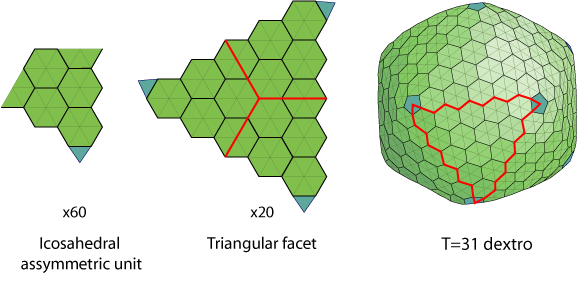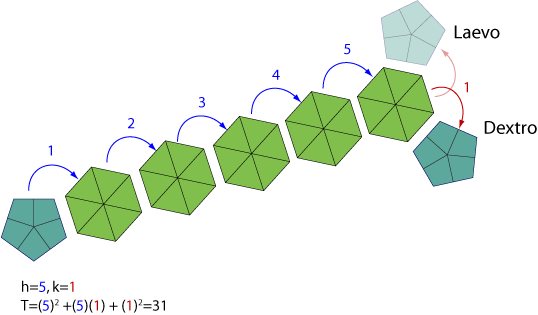# T=31/pseudo T=31 icosahedral capsidThe capsid is composed 60 asymmetric units made of 31 proteins (T=31), for a total of 1860 capsid proteins. These capsids are skewed, and are therefore described as either right- (dextro) or left- (laevo) handed.

Turriviridae capsid symmetry is a pseudo T=31 structure, because hexamers are formed by 3 subunits instead of 6.Icosahedric triangulation number is calculated following Caspar and Klug system